### Home > A2C > Chapter 4 > Lesson 4.1.1 > Problem4-10

4-10.
1. Write the equation for each graph. 4-10 HW eTool (Desmos). Homework Help ✎

1.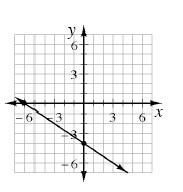2.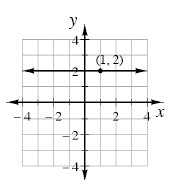3.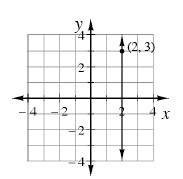4.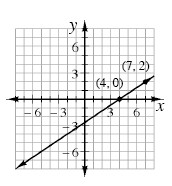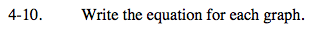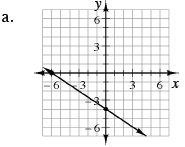What is the y-intercept? Pick two points and determine the slope.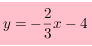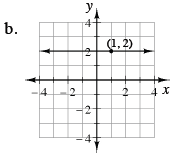Refer to part (a).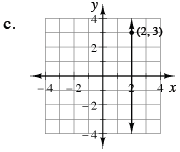Which value (x or y) is equal to 2 on this line? (Look at the point.)

All vertical lines have undefined slope and an equation of the form x = k.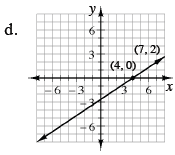Refer to part (a).